# How to Dynamically Extract Unique Values from A Column List in Excel

Suppose we have a list of some objects in one column, and some of them are duplicate, they also can be replaced by typing different object name, here’s the question, how can we dynamically extract unique values from this list in time if they are changed frequently? This article will show you two methods to extract unique values from a dynamic list. If you are good at coding, you can edit VBA code to implement this requirement, if you are weak in coding, you can use some excel functions to extract unique values as well.

## Dynamically Extract Unique Values from A Column List by Formula

Precondition:

Prepare a list of food. You can find that they are duplicate.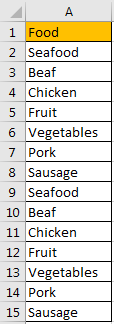Step 1: In any cell except A1:A15, enter the formula =IFERROR(INDEX(\$A\$2:\$A\$15, MATCH(0,COUNTIF(\$C\$1:C1, \$A\$2:\$A\$15), 0)),””).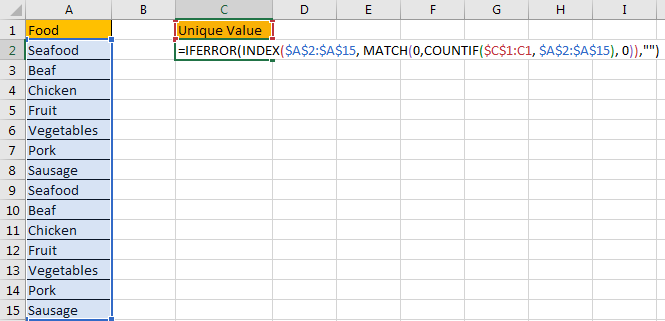Step 2: As it is an array formula, so we need to press Ctrl+Shift+Enter simultaneously to get result.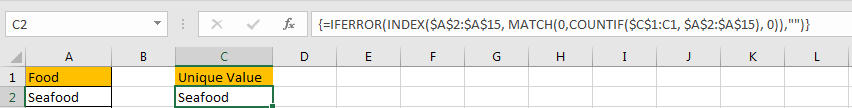We get Seafood in this location.

Step 3: Drag the fill handle down to fill the other cells in C column. Till there is nothing to be shown in cell.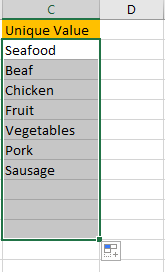So, the list in C column records all unique values from selected range.

Step 4: After above steps, we get all unique values from selected range. Now let’s try to update one value in selected range, and verify if C column can be updated accordingly.

For example, update the second ‘Seafood’ to ‘Orange Juice’.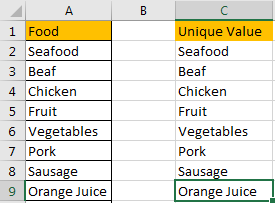Verify that a new value is added in the end of Unique Value list directly. That means above formula works well and applies on C column properly.

## Dynamically Extract Unique Values from A Column List by VBA Code

If you are familiar with coding, you can also through editing VBA code to implement extracting unique values dynamically.

Step 1: On current visible worksheet, right click on sheet name tab to load Sheet management menu. Select View Code, Microsoft Visual Basic for Applications window pops up.

Or you can enter Microsoft Visual Basic for Applications window via Developer->Visual Basic. You can also press Alt + F11 keys simultaneously to open it.

Step 2: In Microsoft Visual Basic for Applications window, click Insert->Module, enter below code in Module1:

```Sub ExtractUniqueValue()

Dim xRng As Range

Dim xRow1 As Long

Dim xRow2 As Long

Dim i As Integer

On Error Resume Next

Set xRng = Application.InputBox("Please select range:", "Extract Unique Value", Selection.Address, , , , , 8)

If xRng Is Nothing Then Exit Sub

On Error Resume Next

xRng.Copy Range("C2")

xRow1 = xRng.Rows.Count + 1

xRow2 = Cells(Rows.Count, "A").End(xlUp).Row

For i = 1 To xLastRow2

If ActiveSheet.Range("C2:C" & xRow2).Cells(i).Value = "" Then

ActiveSheet.Range("C2:C" & xRow2).Cells(i).Delete

End If

Next

End Sub```

Step 3: Save the codes, see screenshot below. And then quit Microsoft Visual Basic for Applications.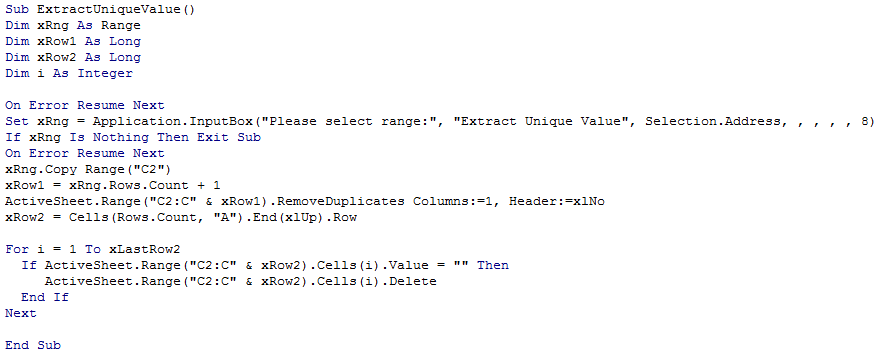Step 4: Click Developer->Macros to run Macro. Select ‘ExtractUniqueValue’ and click Run.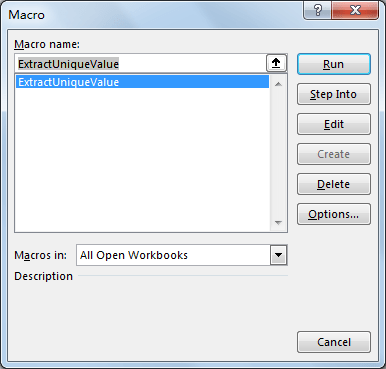Step 5: Extract Unique Value dialog pops up. Enter Select Range \$A\$2:\$A\$15. Click OK. In this step you can select the range you want to extract unique values.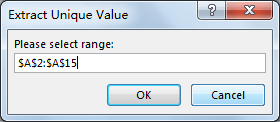Step 6: Click OK and check the result. Verify that unique values are displayed in C column properly. The behavior is as same as the result in method 1 step# 3.

Step 7: If you update the original list, you have to run macro to refresh Unique Value list. This way can also dynamically extract unique values from original list.

### Related Functions

• Excel IFERROR function
The Excel IFERROR function returns an alternate value you specify if a formula results in an error, or returns the result of the formula.The syntax of the IFERROR function is as below:= IFERROR (value, value_if_error)….
• Excel INDEX function
The Excel INDEX function returns a value from a table based on the index (row number and column number)The INDEX function is a build-in function in Microsoft Excel and it is categorized as a Lookup and Reference Function.The syntax of the INDEX function is as below:= INDEX (array, row_num,[column_num])…
• Excel MATCH  function
The Excel MATCH function search a value in an array and returns the position of that item.The MATCH function is a build-in function in Microsoft Excel and it is categorized as a Lookup and Reference Function.The syntax of the MATCH function is as below:= MATCH  (lookup_value, lookup_array, [match_type])….
• Excel COUNTIF function
The Excel COUNTIF function will count the number of cells in a range that meet a given criteria. This function can be used to count the different kinds of cells with number, date, text values, blank, non-blanks, or containing specific characters.etc.= COUNTIF (range, criteria)…

Sidebar